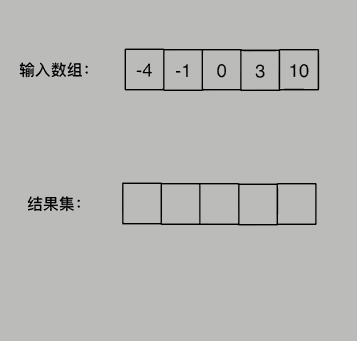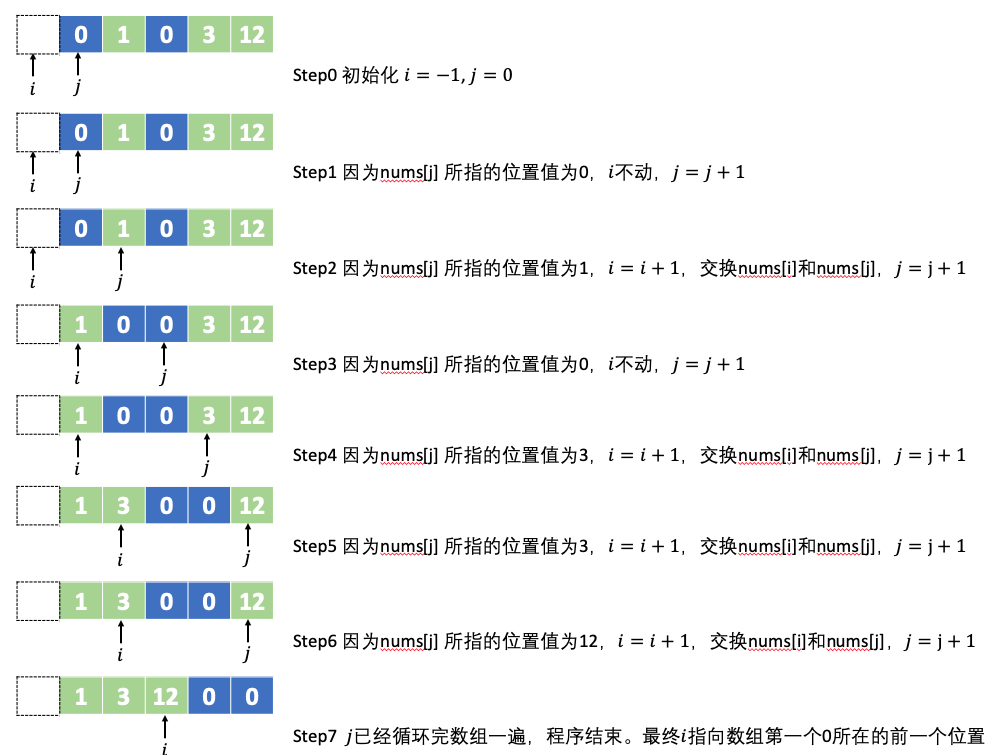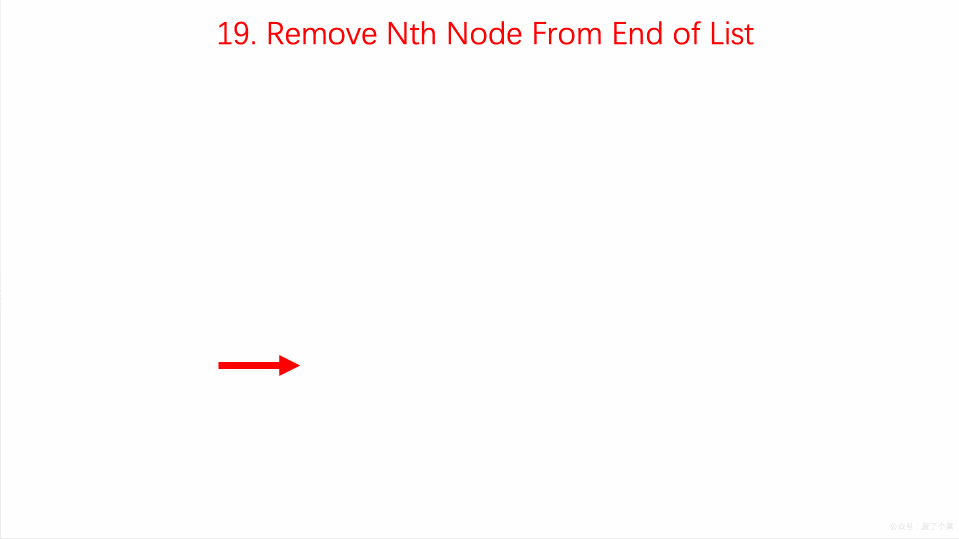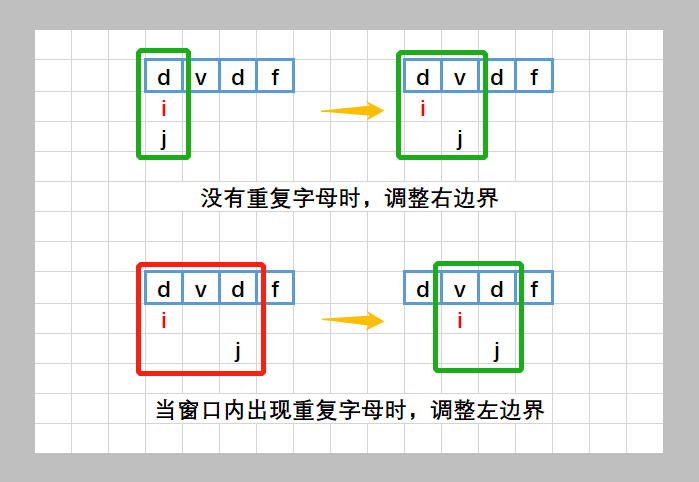# 算法入门

## 二分查找

### 704. 二分查找

#### 题目

输入: nums = [-1,0,3,5,9,12], target = 9

输入: nums = [-1,0,3,5,9,12], target = 2

#### 解答

class Solution:
"""二分查找"""
def search(self, nums: List[int], target: int) -> int:
left, right = 0, len(nums) - 1
while left <= right:
mid = left + ((right -left) >> 1)
if nums[mid] == target:
return mid
elif target < nums[mid]:
right = mid - 1
else:
left = mid + 1
return -1
• 1、循环条件： left <= right
• 循环条件包含了 left == right的情况，则我们必须在每次循环中改变 leftright的指向，以防止进入死循环。
• 2、循环终止条件包括：
• 找到了目标值
• left > right （这种情况发生于当left, mid, right指向同一个数时，这个数还不是目标值，则整个查找结束。）
• 3、中间位置计算： mid = left + ((right -left) >> 1)
• left + ((right -left) >> 1) 其实和 (left + right) / 2是等价的，这样写的目的一个是为了防止 (left + right)出现溢出，用右移操作(>>)替代除法提升了性能。
• 4、左边界更新：left = mid + 1
• 5、右边界更新： right = mid - 1
• 6、返回值： mid / -1

### 278. 第一个错误的版本

#### 题目

输入：n = 5, bad = 4

输入：n = 1, bad = 1

1 <= bad <= n <= 231 - 1

#### 解答

class Solution:
"""
:type n: int
:rtype: int
"""
left, right =1, n
while left < right:
mid = left + ((right -left) >> 1)
right = mid     # 答案在区间 [left, mid] 中
else:
left = mid + 1  # 答案在区间 [mid+1, right] 中
return left             # 此时有 left == right，区间缩为一个点，即为答案

#### 复杂度

• 时间复杂度：$\mathcal{O}(\log n)$​，其中 n 是给定版本的数量。
• 空间复杂度：$\mathcal{O}(1)$。我们只需要常数的空间保存若干变量。

### 35. 搜索插入位置

#### 题目

输入: nums = [1,3,5,6], target = 5

输入: nums = [1,3,5,6], target = 2

输入: nums = [1,3,5,6], target = 7

输入: nums = [1,3,5,6], target = 0

输入: nums = , target = 0

• 1 <= nums.length <= 104
• -104 <= nums[i] <= 104
• nums 为无重复元素的升序排列数组
• -104 <= target <= 104

#### 解答

class Solution:
def searchInsert(self, nums: List[int], target: int) -> int:
left, right = 0, len(nums)- 1
while left <= right:
mid = left + ((right - left) >> 1)
if nums[mid] == target:
return mid
elif target < nums[mid]:
right = mid -1
else:
left = mid + 1
return  left

## 双指针

### 977.有序数组的平方

#### 题目

输入：nums = [-4,-1,0,3,10]

输入：nums = [-7,-3,2,3,11]

• 1 <= nums.length <= 104
• -104 <= nums[i] <= 104
• nums 已按 非递减顺序 排序

#### 解答

class Solution:
def sortedSquares(self, nums: List[int]) -> List[int]:
return sorted(pow(num,2) for num in nuclass Solution:
def sortedSquares(self, nums: List[int]) -> List[int]:
i, j, k = 0, len(nums)-1, len(nums)-1
res = [-1] * len(nums)
while i <= j:
if nums[i] * nums[i] > nums[j] * nums[j]:
res[k] = nums[i] * nums[i]
i += 1
else:
res[k] = nums[j] * nums[j]
j -= 1
k -= 1
return res

### 189. 旋转数组

#### 题目

• 尽可能想出更多的解决方案，至少有三种不同的方法可以解决这个问题。
• 你可以使用空间复杂度为 O(1) 的 原地 算法解决这个问题吗？

输入: nums = [1,2,3,4,5,6,7], k = 3

输入：nums = [-1,-100,3,99], k = 2

• 1 <= nums.length <= 2 * 104
• -231 <= nums[i] <= 231 - 1
• 0 <= k <= 105

#### 解答

class Solution:
def rotate(self, nums: List[int], k: int) -> None:
"""
Do not return anything, modify nums in-place instead.
"""
res = [-1] * len(nums)
for i in range(len(nums)):
res[(i+k)%len(nums)] = nums[i]
for i in range(len(nums)):
nums[i] = res[i]

### 题目

输入: [0,1,0,3,12]

1. 必须在原数组上操作，不能拷贝额外的数组。
2. 尽量减少操作次数。

#### 解答class Solution:
def moveZeroes(self, nums: List[int]) -> None:
"""
Do not return anything, modify nums in-place instead.
"""
i = -1
for j in range(len(nums)):
if nums[j]!=0:
i+=1
nums[i],nums[j]= nums[j], nums[i]


class Solution:
def moveZeroes(self, nums: List[int]) -> None:
"""
Do not return anything, modify nums in-place instead.
"""
n = len(nums)
left = right = 0
while right < n:
if nums[right] != 0:
nums[left], nums[right] = nums[right], nums[left]
left += 1
right += 1

### 167. 两数之和 II - 输入有序数组

#### 题目

输入：numbers = [2,7,11,15], target = 9

输入：numbers = [2,3,4], target = 6

输入：numbers = [-1,0], target = -1

• 2 <= numbers.length <= 3 * 104
• -1000 <= numbers[i] <= 1000
• numbers递增顺序 排列
• -1000 <= target <= 1000
• 仅存在一个有效答案

#### 解答

class Solution:
def twoSum(self, numbers: List[int], target: int) -> List[int]:
left, right = 0, len(numbers) - 1
while left < right:
if numbers[left] + numbers[right] == target:
return [left+1,right+1]
elif numbers[left] + numbers[right] < target:
left += 1
else:
right -= 1
return [-1,-1]

### 复杂度

• 时间复杂度：$\mathcal{O}(n)$​​​​
• 空间复杂度：$\mathcal{O}(1)$​。

### 344. 反转字符串

#### 题目

输入：["h","e","l","l","o"]

输入：["H","a","n","n","a","h"]

#### 解答

class Solution:
def reverseString(self, s: List[str]) -> None:
"""
Do not return anything, modify s in-place instead.
"""
left, right =0, len(s)-1
while left <= right:
s[left],s[right] = s[right],s[left]
left += 1
right -= 1

• s[::-1] 表示反转 s 中的元素
• s[:] 表示数组中所有元素
• s[:]=s[::-1] 表示将原数组反转后赋值给 s 中每一个对应的位置
• s=s[::-1] 表示将 s 反转后赋值给新的对象 s（可以通过id函数查看内存地址），与题意原地修改不符。
class Solution:
def reverseString(self, s: List[str]) -> None:
"""
Do not return anything, modify s in-place instead.
"""
s[:]=s[::-1]

### 557. 反转字符串中的单词 III

#### 题目

输入："Let's take LeetCode contest"

• 在字符串中，每个单词由单个空格分隔，并且字符串中不会有任何额外的空格。

#### 解答

class Solution(object):
def reverseWords(self, s):
return " ".join(word[::-1] for word in s.split(" "))
• s.split(" ") 以空格为分隔符将字符串分割成单词列表

• [::-1] 将单词反转

• " ".join() 将单词列表转换为字符串，以空格分隔

class Solution(object):
def reverseWords(self, s):
return " ".join(s.split(" ")[::-1])[::-1]

• s.split(“ “) 将字符串分割成单词列表:
['I', 'love', 'drag', 'queen']
• s.split(“ “)[::-1] 将单词列表反转:
['queen', 'drag', 'love', 'I']
• “ “.join(s.split(“ “)[::-1]) 将单词列表转换为字符串，以空格分隔:
"queen drag love I"
• “ “.join(s.split(“ “)[::-1])[::-1] 将字符串反转：
”I evol gard neeuq“

## 链表

### 876. 链表的中间结点

#### 题目

输入：[1,2,3,4,5]

ans.val = 3, ans.next.val = 4, ans.next.next.val = 5, 以及 ans.next.next.next = NULL.

输入：[1,2,3,4,5,6]

• 给定链表的结点数介于 1100 之间。

#### 解答

# Definition for singly-linked list.
# class ListNode:
#     def __init__(self, val=0, next=None):
#         self.val = val
#         self.next = next
class Solution:
def middleNode(self, head: ListNode) -> ListNode:
while fast and fast.next:
slow = slow.next
fast = fast.next.next
return slow

### 19. 删除链表的倒数第 N 个结点

#### 题目输入：head = [1,2,3,4,5], n = 2

输入：head = , n = 1

输入：head = [1,2], n = 1

• 链表中结点的数目为 sz
• 1 <= sz <= 30
• 0 <= Node.val <= 100
• 1 <= n <= sz

#### 解答

# Definition for singly-linked list.
# class ListNode:
#     def __init__(self, val=0, next=None):
#         self.val = val
#         self.next = next
class Solution:
def removeNthFromEnd(self, head: ListNode, n: int) -> ListNode:

#step1: 获取链表长度
while cur:
length += 1
cur = cur.next

#step2: 找到倒数第N个节点的前面一个节点
cur = dummy
for i in range(length - n):
cur = cur.next

#step3: 删除节点，并重新连接
cur.next = cur.next.next
return dummy.next 

• 设定双指针 slow 和 fast，初始都指向虚拟节点 dummy
• 移动 fast，直到 slow 与 fast 之间相隔的元素个数为 n
• 再同时移动 slow 与 fast ，直到 fast 指向的为 NULL
• 将 slow 的下一个节点指向下下个节点# Definition for singly-linked list.
# class ListNode:
#     def __init__(self, val=0, next=None):
#         self.val = val
#         self.next = next
class Solution:
def removeNthFromEnd(self, head: ListNode, n: int) -> ListNode:

#step1: 快指针先走n步
slow, fast = dummy, dummy
for i in range(n):
fast = fast.next

#step2: 快慢指针同时走，直到fast指针到达尾部节点，此时slow到达倒数第N个节点的前一个节点
while fast and fast.next:
slow, fast = slow.next, fast.next

#step3: 删除节点，并重新连接
slow.next = slow.next.next
return dummy.next 

## 滑动窗口

### 3. 无重复字符的最长子串# 赞助💰

 支付宝支付 微信支付上一篇Algorithm Review

2021-10-07PyQt 学习记录
PyQt是一个用于创建GUI应用程序的跨平台工具包，它将Python与Qt库融为一体。PyQt允许使用Python语言调用Qt库中的API。这样做的最大好处就是在保留了Qt高运行效率的同时，大大提高了开发效率。 基础第一个 PyQt 程
2021-07-23
目录